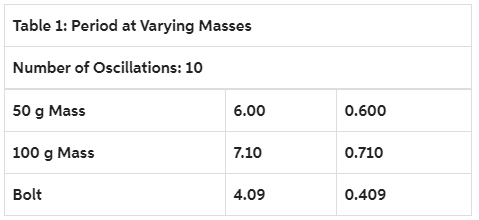# Problem: 1. Describe initial observations about any differences in motion as mass and amplitude changed?Create a plot of the period vs. mass (exclude the steel bolt). Comment on the general shape of your plot and relate this shape to the equation describing SHM:2. Use your data to calculate the spring constant, k, of the spring.Hint: What variables do you need to plot in order to produce a linear graph in order to calculate k?K=F/(x-x0)3. Use the calculated value of k and your data from Part 1: Changing Mass to calculate the mass of the steel bolt.The steel bolt T= 0.409 s  4. Calculate the maximum speed of the masses and steel bolt during the oscillation?Mass of 50 g =Mass of 100 g =Mass of steel bolt =

###### FREE Expert Solution

In this problem, we are applying the concepts of simple harmonic.

This is a laboratory report question. The most important is the concept to solve the problem, not the answers.

Periodic time:

$\overline{){\mathbf{T}}{\mathbf{=}}{\mathbf{2}}{\mathbf{\pi }}\sqrt{\frac{\mathbf{m}}{\mathbf{k}}}}$

96% (457 ratings)###### Problem Details

1. Describe initial observations about any differences in motion as mass and amplitude changed?

Create a plot of the period vs. mass (exclude the steel bolt). Comment on the general shape of your plot and relate this shape to the equation describing SHM:2. Use your data to calculate the spring constant, k, of the spring.

Hint: What variables do you need to plot in order to produce a linear graph in order to calculate k?

K=F/(x-x0)

3. Use the calculated value of k and your data from Part 1: Changing Mass to calculate the mass of the steel bolt.

The steel bolt T= 0.409 s

4. Calculate the maximum speed of the masses and steel bolt during the oscillation?

Mass of 50 g =

Mass of 100 g =

Mass of steel bolt =